0
78

# Height and Distance Questions for SSC CHSL set-2 PDF

##### Download SSC CHSL Height and Distance questions with answers Set-2 PDF based on previous papers very useful for SSC CHSL Exams. Top-15 Very Important Height and Distance Questions for SSC Exam

Question 1: From an aeroplane just over a straight road, the angles of depression of two consecutive kilometre stones situated at opposite sides of the aeroplane were found to be 60° and 30° respectively. The height (in km) of the aeroplane from the road at that instant was (Given √3 = 1.732)

a) 0.433

b) 8.66

c) 4.33

d) 0.866

Question 2: A person of height 6ft. wants to pluck a fruit which is on a 26/3 ft. high tree. If the person is standing 8/√3 ft. away from the base of the tree, then at what angle should he throw a stone so that it hits the fruit ?

a) 75°

b) 30°

c) 45°

d) 60°

Question 3: The angle of elevation of a tower from a distance of 100 metre from its foot is 30°. Then the height of the tower is

a) $50\sqrt{3}$ metre

b) $100\sqrt{3}$ metre

c) $\frac{50}{\sqrt{3}}$ metre

d) $\frac{100}{\sqrt{3}}$ metre

Question 4: A kite is flying at the height of 75m from the ground. The string makes an angle θ (where cotθ = 8/15) with the level ground. Assuming that there is no slack in the string the length of the string is equal to :

a) 85 metre

b) 65 metre

c) 75 metre

d) 40 metre

Question 5: The angle of elevation of the top of a vertical tower situated perpendicularly on a plane is observed as 60° from a point P on the same plane. From another point Q, 10m vertically above the point P, the angle of depression of the foot of the tower is 30°. The height of the tower is

a) 15 m

b) 30 m

c) 20 m

d) 25 m

SSC CHSL Study Material (FREE Tests)

Question 6: From a point 20 m away from the foot of a tower, the angle of elevation of the top of the tower is 30°. The height of the tower is

a) 10 √3 m

b) 20 √3 m

c) $\frac{10}{\sqrt{3}}$ m

d) $\frac{20}{\sqrt{3}}$ m

Question 7: The length of the shadow of a tower is 9 metres when the sun’s altitude is 30°. What is the height of the tower ?

a) $3\sqrt{3}$ m

b) $4\frac{1}{2}$ m

c) $9\sqrt{3}$ m

d) $\frac{9\sqrt{3}}{2}$ m

Question 8: A right pyramid 6 m height has a square base of which the diagonal is $\sqrt{1152} m$. Volume of the pyramid is

a) 144 m

b) 288 m

c) 576 m

d) 1152 m

Question 9: From the top of a cliff 90 metre high, the angles of depression of the top and bottom of a tower are observed to be 30° and 60° respectively. The height of the tower is :

a) 45 m

b) 60 m

c) 75 m

d) 30 m

Question 10: A boy standing in the middle of a field, observes a flying bird in the north at an angle of elevation of 30° and after 2 minutes, he observes the same bird in the south at an angle of elevation of 60°. If the bird flies all along in a straight line at a height of 50 m, then its speed in km/h is :

a) 4.5

b) 3

c) 9

d) 6

Question 11: A tree is broken by the wind. If the top of the tree struck the ground at an angle of 30° and at a distance of 30 m from the root, then the height of the tree is

a) 25√3 m

b) 30√3 m

c) 15√3 m

d) 20√3 m

Question 12: At an instant, the length of the shadow of a pole is , √3 times the height of the pole. The angle of elevation of the Sun at that moment is

a) 75°

b) 30°

c) 45°

d) 60°

Question 13: The height of a tower is h and the angle of elevation of the top of the tower is a. On moving a distance h/2 towards, the tower, the angle of elevation becomes 0. The value of cotα – cot β is

a) 1

b) 2

c) 1/2

d) 2/3

Question 14: If the angles of elevation of a balloon from two consecutive kilometre-stones along a road are 30° and 60° respectively, then the height of the balloon above the
ground will be

a) $\frac{\sqrt{3}}{2}$ km

b) $\frac{1}{2}$ km

c) $\frac{2}{\sqrt{3}}$ km

d) $3\sqrt{3}$

Question 15: A tower is broken at a point P above the ground. The top of the tower makes an angle 60° with the ground at Q. From another point R on the opposite side of Q angle of elevation of point P is 30°. If QR = 180 m, then what is the total height (in metres) of the tower?

a) $90$

b) $45\sqrt{3}$

c) $45(\sqrt{3}+1)$

d) $45(\sqrt{3}+2)$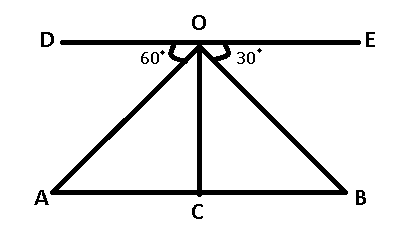OC = height of plane = $h$

$\angle$OAC = $\angle$DOA = 60°

$\angle$OBC = $\angle$BOE = 30°

AB = 2 and let AC = $x$

=> BC = $(2-x)$

From, $\triangle$OAC

$tan60^{\circ} = \frac{OC}{AC}$

=> $\sqrt{3} = \frac{h}{x}$

=> $x = \frac{h}{\sqrt{3}}$ ————Eqn(1)

From, $\triangle$OBC

$tan30^{\circ} = \frac{OC}{BC}$

=> $\frac{1}{\sqrt{3}} = \frac{h}{2-x}$

=> $\sqrt{3}h = 2 – \frac{h}{\sqrt{3}}$ [From eqn(1)]

=> $\frac{3h+h}{\sqrt{3}} = 2$

=> $h = \frac{2\sqrt{3}}{4} = \frac{\sqrt{3}}{2}$

= $\frac{1.732}{2}$ = 0.866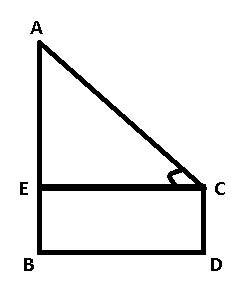Height of person = CD = 6 ft

Height of tree = AB = $\frac{26}{3}$ ft

Distance between them = BD = $\frac{8}{\sqrt{3}}$ ft

To find : $\angle$ACE = $\theta$ = ?

Solution : AE = AB – BE = $\frac{26}{3}$ – 6

=> AE = $\frac{8}{3} ft$

and BD = CE = $\frac{8}{\sqrt{3}}$ ft

Now, in $\triangle$AEC

=> $tan\theta$ = $\frac{AE}{CE}$

=> $tan\theta$ = $\frac{\frac{8}{3}}{\frac{8}{\sqrt{3}}}$

=> $tan\theta$ = $\frac{1}{\sqrt{3}}$

=> $\theta$ = 30°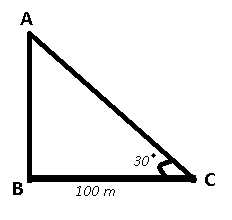Height of tower = AB

In $\triangle$ABC

=> $tan\theta = \frac{AB}{BC}$

=> $tan30^{\circ} = \frac{AB}{100}$

=> $\frac{1}{\sqrt{3}} = \frac{AB}{100}$

=> $AB = \frac{100}{\sqrt{3}}$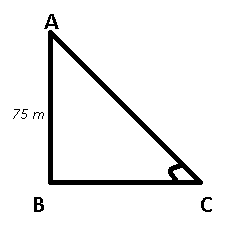Height of kite from ground = AB = 75 m

$\angle$ACB = $\theta$

We know that $cot\theta = \frac{8}{15}$

=> $\frac{BC}{AB} = \frac{8}{15}$

=> $BC = \frac{8*75}{15} = 40 m$

Now, length of string AC = $\sqrt{(AB)^2 + (BC)^2}$

=> AC = $\sqrt{75^2 + 40^2}$

= $\sqrt{5625+1600} = \sqrt{7225}$

=> AC = 85 m

In the given problem, distance between tower and point of observation will be the adjacent side and tower will be the opposite side.
Let ‘d’ be the distance and ‘h’ be the height of the tower.
tan 60 = h/d => d = h/tan 30
tan 30 = (h-10)/d => d = (h-10)/ tan 60
h/tan 30 = (h-10)/tan 60
h/h-10 = 3
2h = 30
h = 15m
Option A is the correct answer.

Perpendicular AB= height of tower

Base BC = distance b/w base of tower and the point

Hypotenuse AC= segment b/w top of the tower and the point

Angle of elevation ACB =30°

tan 30° = Perpendicular / Base = 1/√3

Perpendicular = Base*1/√3

Base is 20 m.

So height of tower is 20/√3m.

Height of tower will be the opposite side.
Hence, tan 30 = height/length
$1/\sqrt{3}$ = $h/9$
=>$h = 3\sqrt{3}$
Option A is the right answer.

It is given that base of pyramid is square and diagonal is given as $\sqrt{1152} m$

we know diagonal of sqaure is $\sqrt{2}$ a , where a is the side of sqaure

so, $\sqrt{1152} m$ = $\sqrt{2} a$

a = 24 m

Area of base = $\text{side}^2$ = $24^2$ = 576

Height of pyramid = 6 m

Volume of right pyramid = $\frac{1}{3} {\times \text{Base Area} \times \text{Height}}$ = $\frac{1}{3} \times 576 \times 6$ = $1152m^{3}$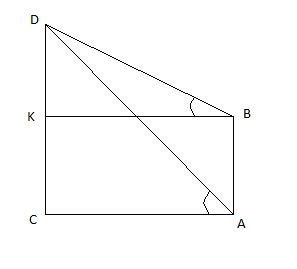Given: CD = 90 meter;
∠KBD = 30° & ∠CAD = 60°
BD × cos30° = BK
BD × sin30° = DK
BK = AC
BD × cos30° = AD × cos60°
√ 3 × BD = AD ____________ (1)
Now, DC = DK + KC
DC – DK = AB [As, KC = AB]
(AD × sin60°) – (BD × sin30°) = AB
Using equation (1)
($\sqrt{3}$ ×BD×$\frac{\sqrt{3}}{2}$)BD2=AB

(3×BD×32)−BD2=AB

AB = BD _____________ (2)
Now, AD × sin 60° = DC = 90 meter
AD=$\frac{180}{\sqrt{3}}$

From (1)
BD=AD/$\sqrt{3}$

=180/$\sqrt{3} \times \sqrt{3}$ = 60 meter

From (2) AB = 60 meter
Option B is the correct answer.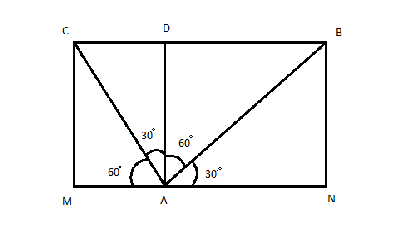From the diagram,
Height = AD = 50√3 m
∠BAN = 30°
∠CAM = 60°
∴∠BAD = 90° – 30° = 60°
∴∠CAD = 90° – 60° = 30°
From ΔABD,
√3 = BD/(50√3)
BD = 50 × 3 = 150 m
From ΔACD,
1/√3 = CD/(50√3)
CD = 50 m
∴ Distance travelled by the bird
= BC = BD + CD = 150 m + 50 m = 200 m = 0.200 km
Time taken to cover this distance = 2 minutes = 2/60 hr = 1/30 hr
∴ Speed
= Distance travelled/ Time required
=0.200 $\times$30km/hr

= 0.200 × 30 km/hr
= 6 km/hr
Option D is the correct answer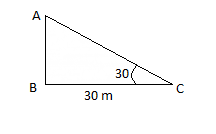$tan 30 = \frac{AB}{BC}$ =$\frac{1}{\sqrt{3}}$
$cos 30 = \frac{BC}{AC} = \frac{sqrt{3}}{2}$
Height of the tree = $AB + AC$
$AB= BC \times \frac{1}{\sqrt{3}}$
$AB= 30 \times \frac{1}{\sqrt{3}} = \frac{30}{\sqrt{3}}$
$AC = \frac {2 \times BC}{\sqrt{3}} =\frac {2 \times 30}{\sqrt{3}}$
$AB+AC = \frac{30}{\sqrt{3}} + \frac {60}{\sqrt{3}} = 30\sqrt{3}$
Hence Option B is the correct answer.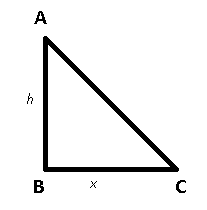Let height of pole = AB = $h$

Length of shadow = BC = $x$

Angle of elevation of sun = $\angle$ACB = $\theta$ = ?

Acc to ques : $x = \sqrt{3} h$

In $\triangle$ABC

=> $tan \theta = \frac{AB}{BC}$

=> $tan \theta = \frac{h}{x}$

=> $tan \theta = \frac{h}{\sqrt{3} h} = \frac{1}{\sqrt{3}}$

=> $\theta$ = 30°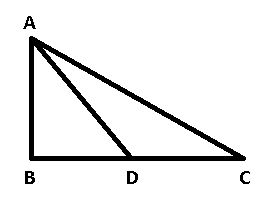Here, $\angle$ACB = $\alpha$ and $\angle$ADB = $\beta$

AB = tower = $h$ metre

and CD = $\frac{h}{2}$ metre

From $\triangle$ABC

=> $tan \alpha = \frac{AB}{BC} = \frac{h}{BC}$

=> $BC = h cot \alpha$ ———-Eqn(1)

From $\triangle$ABD

=> $tan \beta = \frac{AB}{BD} = \frac{h}{BC – CD}$

=> $tan \beta = \frac{h}{h cot \alpha – \frac{h}{2}}$

=> $h cot \alpha – \frac{h}{2} = h cot \beta$

=> $h (cot \alpha – cot \beta) = \frac{h}{2}$

=> $cot \alpha – cot \beta = \frac{1}{2}$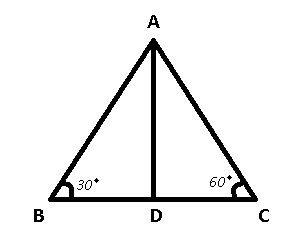It is given that BC = 2 km

Let BD = x => CD = (2 – x) km

From $\triangle$ABD

=> $tan 30 = \frac{AD}{BD}$

=> $\frac{1}{\sqrt{3}} = \frac{AD}{x}$

=> $AD = \frac{x}{\sqrt{3}}$

From $\triangle$ADC

=> $tan 60 = \frac{AD}{CD}$

=> $\sqrt{3} = \frac{\frac{x}{\sqrt{3}}}{2 – x}$

=> $3 (2 – x) = x$

=> $x = \frac{3}{2}$

Now, height of balloon above ground = $AD = \frac{\frac{3}{2}}{\sqrt{3}}$

= $\frac{\sqrt{3}}{2}$ km

In $\triangle$ PRS,

=> $tan(30^\circ)=\frac{PS}{RS}$

=> $\frac{1}{\sqrt3}=\frac{x}{d}$

=> $d=\sqrt3x$ ————(i)

Similarly, in $\triangle$ PQS,

=> $tan(60^\circ)=\frac{PS}{SQ}$

=> $\sqrt3=\frac{x}{180-d}$

=> $180\sqrt3-3x=x$     [Using equation (i)]

=> $x+3x=4x=180\sqrt3$

=> $x=\frac{180\sqrt3}{4}=45\sqrt3$ ————(ii)

Again, in $\triangle$ PQS,

=> $sin(60^\circ)=\frac{PS}{PQ}$

=> $\frac{\sqrt3}{2}=\frac{x}{y}$

=> $\sqrt3y=2(45\sqrt3)$       [Using equation (ii)]

=> $y=\frac{90\sqrt3}{\sqrt3}=90$ ———–(iii)

Adding equations (ii) and (iii), we get :

=> $x+y=45\sqrt3+90$

=> Height of tower = $45(\sqrt3+2)$ m

=> Ans – (D)

We hope this Height and Distance  questions of set-2 pdf for SSC CHSL Exam preparation is so helpful to you.